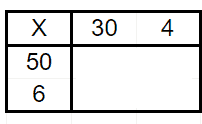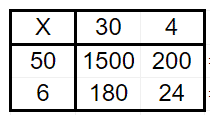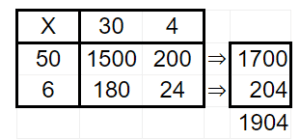# Box Method Multiplication Worksheets

• ###### Box Method Multiplication #15

These worksheets were created to help students practice and master the box method or grid method for multiplication. The box method is an alternative approach to traditional long multiplication and provides a visual representation of the multiplication process.

In box method multiplication, a grid or box is created to organize the multiplication of two or more numbers. The grid is divided into rows and columns, with each cell representing a partial product. The digits of the multiplicand and the multiplier are placed in the corresponding cells of the grid, and then the products of each digit pair are calculated and added up to find the final product.

Here’s an example of how the box method works:

Let’s multiply 34 by 56 using the box method.

Step 1: Create a grid with two rows and two columns.Step 2: Multiply the digits in each cell of the grid.Step 3: Add up the values in each column.Step 4: The final product is 1,904.

Box method multiplication worksheets provide students with exercises that involve various numbers to multiply. They typically include partially filled grids for students to complete and practice the box method. The worksheets can be progressively more complex, introducing larger numbers and multi-digit factors.

By using box method multiplication worksheets, students can develop their understanding of multiplication concepts, improve their problem-solving skills, enhance their ability to visualize the multiplication process, and strengthen their overall mathematical proficiency.

### Why Use This Technique Over Others?

The box method or grid method for multiplication offers several advantages over traditional long multiplication or other methods. Here are some reasons why the box method may be preferred:

Visual With Place Value Emphasis

The box method provides a visual representation of the multiplication process. The grid allows students to see the organization of digits, partial products, and the overall structure of the multiplication. This visual aid can help students better understand the concept of multiplication and how the digits interact with each other.

The box method highlights the importance of place value in multiplication. Each cell in the grid represents a specific place value, making it easier for students to understand the significance of each digit’s position. This emphasis on place value can deepen students’ understanding of the number system and strengthen their overall number sense.